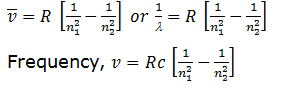# Which of the following transitions in a hydrogen atom emits the photon of highest frequency?         (a)    n = 2 to n = 1         (b)    n = 2 to n = 6         (c)    n = 6 to n = 2    (d)    n = 1 to n = 2

9 years ago

(a)Note: See the greatest energy difference and also see that the transition is from higher to lower energy level. Hence, it is highest in case of n = 2 to n = 1.

2 years ago
Dear Student,